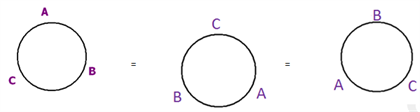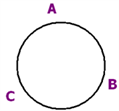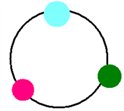# Permutation Questions

Permutation: Permutation is defined as the arrangement of r things that can be done out of total n things.

Formula of Permuation

Permutation is denoted by  nPr   it is equal to  n!/(n-r)!

nPr = n!/(n-r)!

Arrangement of n things at n places = nPn = n!

This is nothing but an arrangement of n things among themselves or can also be written as an arrangement of n things taken all at a time.

Permutation can be divided into four types

1.2.1) Permutation where some items are identical

1.2.2) Permutation with repetitions

1.2.3) Circular permutations

1.2.4) Permutation with restrictions

We will name these four types as

P TYPE 1,

P TYPE 2,

P TYPE 3  and

P TYPE 4  respectively.

1.2.1  P TYPE 1

Number of arrangements of N things among themselves out of which ‘P’ are of one kind, ‘Q’ of another kind, ‘R’ of the third kind;

and the rest all different = N!/(P! Q! R!)

Question 1) How many 3 letter words can be formed from the letters A,A,B?

Solution:

Suppose the letters were A, B, C, instead of A, A, B; then we know the answer will be 3P3 = 3 != 6 ways.

Manually ABC, ACB, BAC, BCA, CAB, CBA are the 6 cases.

But when two of the letters are identical (A,A);

Total number of cases will only be N!/P! (As P things are identical and of the same type)= 3!/2! ways = 3 ways.

Manually solving, AAB, ABA, BAA are the 3 ways.

Illustration:

Question 2) In how many ways can you arrange 4 similar apples, 5 similar oranges and 3 similar pears in a row?

Solution:

This is a PTYPE 1 question where N= 4+5+3 =12 .

Here the total number of arrangements= N!/(P! Q! R!)=12!/(4!*5!*3!)= 27720

Question 3) In how many ways can all the letters of the word ‘CONSTANTINOPLE’ be arranged such that these begin with two T’s and end with S?

Solution:

This is a permutation type 1 question. CONSTANTINOPLE has 3 Ns, 2 Ts, 2 Os and one each of C, S, A ,I, P, E and L

Be sure to note the additional restriction given in the question that says that it should start with 2 Ts and end with an S.

This can be done in only 1 way Now, there are 14-3=11 letters left to be arranged, which include 3 N and 2 O These 11 letters can be arranged in 11!/(3! 2!) ways, which is the answer

1.2.2 P TYPE 2:

Permutation with Repetitions

General formula: Nx

Consider cases where repetitions are allowed. For example, when there are 5 postboxes and 4 letters.

The number of ways in which the letters can be put into the post boxes will be 54

This also can be easily explained using FPC.

1) First letter can be posted in any of the 5 post boxes, thus 5 ways

2) In the same way the 2nd letter can also be posted in any of the post boxes in 5 ways,

3) 3rd letter in 5 ways and the 4th letter also in 5 ways.

That means total no of arrangements will be 5x5x5x5 = 54 ways.

Note: The answer is not 45 but it is 54. This is because, the process of selection of letters which will go into the post boxes is mutually independent, but the process of selection of post boxes into which the letters will go are dependent on each other.

Similarly, consider 3 rings that need to be put into 5 fingers. The number of ways of inserting the rings= 5x5x5=53

(i.e; the first ring can go into any of the 5 fingers, the second ring can also go into any of the five fingers and same for the third ring)

ILLUSTRATIONS

Question 4) In how many ways can 5 prizes be distributed among 4 boys when every boy can win zero or more prizes?

Solution:

If every boy can win zero or more prizes, this question becomes a permutation with repetition question.

Thus, the number of distributions is 45 or 210=1024

1.2.3  P TYPE 3:

CIRCULAR PERMUTATION

General formula (n-1)!

Take a case of 3 persons A, B and C. They can be arranged in a line in 3!=6 ways as given below

1) ABC

2) BCA

3) CAB

4) ACB

5) CBA

6) BAC

This is linear arrangement. Now when you arrange them around a circle, you won’t be able to distinguish between ABC, BCA and CAB.

These 3 cases of linear arrangement will be represented by one circular arrangement.

Similarly, ACB, CBA and BCA can be represented by a single case.ACB, CBA, BAC can’t be distinguished.

Similarly, ABC, BCA, CAB can’t be distinguished.

Thus, it can be seen that in this case for every three linear arrangements there is only 1 circular arrangement.

Therefore the number of circular arrangements will be (number of linear arrangements)/3=3!/3 Hence the total number of arrangements reduces to (3-1)! =2!. The total cases are hence only 2.

Another way of looking at circular arrangement is:- “Fixing of one person and then the linear arrangement of the remaining people.”

For e.g. for 3 people, fix the position of one of them. Let us fix the position of “A”Now we will arrange the remaining two people? B&C = 2! Ways

Generalizing,

If you want to seat N people around a circular table, the number of ways of doing that will be (N-1)! , because N!/N= (N-1)!

A SPECIAL CASE

We human beings, are not symmetric from top to bottom, i.e.

the view is different when you look at a person from an aeroplane and when you look at him from below. However,

if you take a case of differently coloured beads in a necklace,

it will be symmetric from top to bottom.In other words, a certain arrangement and its mirror image is one and the same, while for human beings; it is 2 separate cases.

Hence we will be double counting the cases if we take it as (n-1)!

The number of arrangements will actually be (n-1)!/2.

Hence P TYPE 3 can be summarized as follows

Number of ways in which ‘N’ things can be arranged around a circle if the objects are not

symmetric from top to bottom = (N – 1)!

NUMBER OF WAYS IN WHICH ‘N’ THINGS CAN BE ARRANGED AROUND A CIRCLE IF THE OBJECTS ARE

Number of ways in which ‘N’ things can be arranged around a circle if the objects are

symmetric from top to bottom = (N – 1)!/2

Illustrations

Question 5) The number of ways of arranging ‘x’ different beads to form a necklace is 20160.

Then the number of ways of arranging 2x flowers to form a garland is a) 2x! b) ½(17!) c) 14! d) ½ (13!)

Solution:

From the data in the question, we know that (x-1)1/2=20160 (Symmetric Circular Permutation)

From trial and error, we can obtain the value of x=9 To arrange 2x flowers to form a garland = No of ways of arrangement of 2 x (9)=18 flowers = (18-1)1/2=17!/2

1.2.4  P TYPE 4:

Permutation with restrictions We will solve this type of question in two steps.

i) We will first do the selection in nCr ways.

ii) We will then take one selection and find the number of arrangements possible for that single selection.

Suppose number of arrangements possible = N Then, total number of permutations possible = N x nCr

ways. You will understand it easily with the help of a few illustrations:

Illustration

Question 6) In how many ways can you colour a flag with 4 vertical stripes using the 7 rainbow colours?

a) When there are no restrictions?

b) When indigo and blue are always selected?

c) When indigo and blue are never included?

d) Repetition is allowed but adjacent strips should not be of the same color.

Solution:

a) When there are no restrictions, number of ways = 7P4

b) Now we have a restriction that indigo and blue are always chosen.

Step (i) First make the selection. In this case, two colours are already selected. Select the remaining two colours from the 5 colours in 5C2 ways.

Step (ii) Now do the arrangement. The 4 colours on the flag can be arranged in 4! Ways. The total number of arrangements are thus, (5C2)x4! c)

Step (i) As indigo and blue are never included; the 4 colours are chosen from the remaining 5 colours.

This can be done in 5C4 ways.

Step (ii) The number of ways of arranging the 4 colours will be 4! Hence the answer is (5C4)x4!

d) The colour of the first strip can be chosen in 7 ways, the second in 6 ways (because we should choose a colour which is not there in the first strip), the third in 6 and the fourth also in 6 ways. Thus the total number of ways=7×63

Question 7) The number of ways that 7 persons A,B,C,D,E,F,G can address a meeting so that A will speak before B and B before C is

Solution:

Note in this question that it is the slots for speaking rather than the speakers that need to be selected.

We can solve the question using the following

steps

1) Make the selection first.

2) Assume any one selection manually

3) For that particular selection,

find the number of arrangements

1) Slots for A,B, and C can be selected in 7C3 Ways.

2) Assume that slots 2,4,5 are selected.

3) Now for this particular selection, A,B,C can be arranged in only one way so that the condition that A will speak before B and B will speak before C is satisfied. 4) The other 4 slots can be arranged in 4! Ways. Thus the total number of arrangements are 7C3x4! =840

Question 8) How many 3-digit numbers can be formed using 1,2….,9 such that the hundred’s place is greater than the ten’s place is greater than the unit’s place?

a) 45

b) 108

c) 84

d) 96

Solution:

The question can be solved using the following three steps

1) Make the selection first.

2) Assume any one selection manually

3) For that particular selection,

find the number of arrangements In this question

1) Select 3 digits out of 9 numbers in 9C3 ways

2) For a particular selection, check how many arrangements are possible

Example) if the selection is 9, 5 and 6; then the arrangement such that hundred’s place is greater than ten’s place, which is greater than unit’s place can be done in only one way as 9 6 5.

Thus for any selection there is only one arrangement

3) The answer will be 9C3x1 = 9C3.=84